## Friday, February 26, 2010

### 90

90 = 2 x 32 x 5.

90 has a representation as a sum of two squares: 90 = 32 + 92.

90 is the smallest number that has six representations as a sum of four positive squares: 90 = 12 + 22 + 22 + 92 = 12 + 22 + 62 + 72 = 12 + 32 + 42 + 82 = 22 + 52 + 52 + 62 = 32 + 32 + 62 + 62 = 32 + 42 + 42 + 72.

90 is 66 in base 14.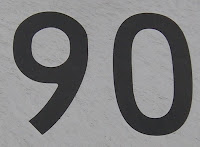As a compass direction, 90 degrees corresponds to east.

Source: WolframAlpha

## Thursday, February 25, 2010

### 7209

7209 = 34 x 89.

7209 has a representation as a sum of two squares: 7209 = 452 + 722.

7209 has a fourth power that is the sum of four fourth powers.

7209 is 30006 in base 7, 10800 in base 9, and 1018 in base 19.LEGO set 7209, introduced in 2004, featured a pteranodon.

## Wednesday, February 24, 2010

### 3974

3974 = 2 x 1987.

3974 = 302 + 312 + 322 + 332.

In its base 5 representation, 3974 has exactly three 1s and two 4s: 111344.

3974 has exactly nine distinct permutations that give primes: 3947, 4397, 4793, 4937, 4973, 7349, 9437, 9473, and 9743.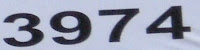3974 Verveer is a main-belt asteroid discovered in 1982.

## Tuesday, February 23, 2010

### 8898

8898 = 2 x 3 x 1483.

8898 is the number of binary strings of length 18 such that no subsequence of length 5 or less contains three or more ones.

8898 is the sum of all composite numbers less than the 35th prime number.

8898 is the number of traces of a 17-step random walk on a one-dimensional lattice, ignoring translations.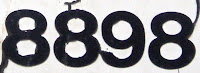8898 Linnaea is an main-belt asteroid discovered in 1995.

## Monday, February 22, 2010

### 2658

2658 = 2 x 3 x 443.

2658 has the representation 25 x 34 + 66.

26581 + 26582 + 26583 + 26584 + 1 and 26581 + 26582 + 26583 + 26584 - 1 are twin primes.

2658 is a square-free number and its neighbors, 2657 and 2659, are both primes.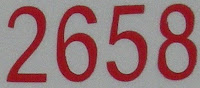NGC 2658 is an open cluster in the constellation Pyxis.

## Friday, February 19, 2010

### 2833

2833 is a prime number.

2833 has a representation as a sum of two squares: 2833 = 232 + 482.

2833 is the hypotenuse of a primitive Pythagorean triple: 28332 = 17752 + 22082.

32833 - 22833 is a prime number.

2833 is a number such that each digit leaves the same nonzero remainder when each is divided into the number.NGC 2833 is a galaxy in the constellation Lynx.

## Thursday, February 18, 2010

### 4112

4112 = 24 x 257.

4112 has a representation as a sum of two squares: 4112 = 42 + 642.

4112 has a representation as sum of two fourth powers: 4112 = 24 + 84.

4112 is the number of necklaces possible with 17 beads, each being one of two colors.

4112 is 1000000010000 in base 2 (binary), 1000100 in base 4, 112422 in base 5, 10020 in base 8, and 1010 in base 16.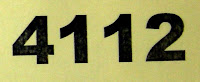NGC 4112 is a galaxy in the constellation Centaurus.

## Wednesday, February 17, 2010

### 1144

1144 = 23 x 11 x 13.

1144 is the number of non-invertible knots with 12 crossings.

1144 is 3223 in base 7.

1144 is a multiple of 11 containing 11 in its decimal representation.

1144 is the number of different necklaces with 7 black beads and n - 7 white beads, where n = 10.1144 Oda is a main belt asteroid discovered in 1930.

## Tuesday, February 16, 2010

### 9031

9031 = 11 x 821.

9031 is a number that cannot be written as a sum of three squares.

9031 is 13344 in base 9 and 3411 in base 14.

9031 is the difference of two consecutive, nonnegative fifth powers: 75 - 65 = 9031.

9031 is the sum of seven distinct powers of three.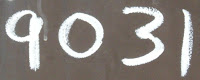NGC 891, also designated PGC 9031, is an edge-on, unbarred spiral galaxy about 30 million light-years away in the constellation Andromeda.

## Monday, February 15, 2010

### 353

353 is a prime number.

353 has a representation as a sum of two squares: 353 = 82 + 172.

353 is the hypotenuse of a primitive Pythagorean triple: 3532 = 2252 + 2722.

353 is the smallest multidigit palindromic prime whose digits are all prime numbers.

666 is the sum of two consecutive palindromic primes: 313 + 353 = 666.

353 is the smallest prime for which its fourth power can be written as the sum of four integers to the fourth power: 3534 = 30 4 + 120 4 + 272 4 + 315 4.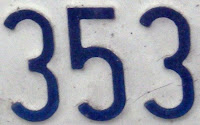353 Ruperto-Carola is a small asteroid discovered by Max Wolf in 1893 in Heidelberg.

## Friday, February 12, 2010

### 570

570 = 2 x 3 x 5 x 19.

570 is the product of the only four prime Roman numeral palindromes: 570 = II x III x V x XIX.

570 is a triangular matchstick number, 3n(n + 1)/2 for n = 19.

570 is the perimeter of a Pythagorean triple: 570 = 95 + 228 + 247 and 2472 = 952 + 2282.Muhammad, founder of the Islamic religion, was born in the year 570 in the city of Mecca.

## Thursday, February 11, 2010

### 985

985 = 5 x 197.

985 has two representations as a sum of two squares: 985 = 122 + 292 = 162 + 272.

985 is the hypotenuse of two primitive Pythagorean triples: 9852 = 4732 + 8642 = 6962 + 6972. It is the fourth Pythagorean triple where two sides are consecutive integers.

985 is the ninth Pell number.985 is the telephone area code for southeastern Louisiana.

## Wednesday, February 10, 2010

### 839

839 is a prime number.

(839 - 8)8 + (839 - 3)3 + (839 - 9)9 is a prime.

839 is a number that cannot be written as a sum of three squares.

839 has a base 5 representation (11324) that begins with its base 9 representation (1132).

839 is 31013 in base 4.Charles the Fat, Holy Roman Emperor, was born in the year 839.

## Tuesday, February 9, 2010

### 284

284 = 22 x 71.

284 is a number that cannot be written as a sum of three squares.

284 = 28 + 28.

284 and 220 form a pair of amicable numbers, the smallest amicable pair. Two numbers n and m are called an amicable pair if the sum of all positive divisors of n is equal to the sum of all positive divisors of m and both are equal to n + m.The Greek mathematician Diophantus died in the year 284.

## Monday, February 8, 2010

### 792

792 = 23 x 32 x 11.

792 is 1100011000 in base 2 (binary) and 2211 in base 7.

792 is the number of integer partitions of 21.

792 is a number that can be expressed as the difference of the squares of primes in exactly four distinct ways.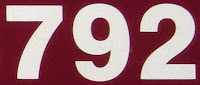792 is the number of inches in a chain, a unit used in surveying.1 mile = 8 furlongs = 80 chains = 5280 feet = 8000 links = 63,360 inches.

Source: Numeropedia

## Friday, February 5, 2010

### 925

925 = 52 x 37.

925 has three representations as a sum of two squares: 925 = 52 + 302 = 142 + 272 = 212 + 222.

925 is the hypotenuse of two primitive Pythagorean triples: 9252 = 432 + 9242 = 5332 + 7562.

925 is 1021021 in base 3 and 4141 in base 6.